# 1.8 新式类和经典类的区别？¶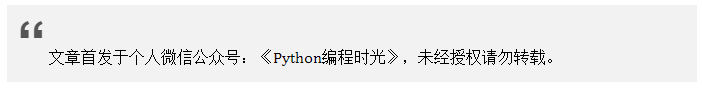## 1. 版本支持 / 写法差异¶

# 不继承自object
class Ming:
pass

# 显示继承object
class Ming(object):
pass


class Ming:
pass

class Ming():
pass

class Ming(object):
pass


## 2. 使用方法 / 独特属性¶

# 经典类：py2.7
class Kls01:
def __getattribute__(self, *args, **kwargs):
print("MING - 01")

# 新式类
class Kls02(object):
def __getattribute__(self, *args, **kwargs):
print("MING - 02")

kls02 = Kls02()
kls02.name

kls01 = Kls01()
kls01.name


MING - 02
Traceback (most recent call last):
File "F:/Python Script/Tornado/yang.py", line 13, in <module>
kls01.name
AttributeError: Kls01 instance has no attribute 'name'


## 3. MRO 查找算法的演变¶

import inspect

class A:pass
class B(A):pass
class C(A):pass
class D(B, C):pass

print inspect.getmro(D)


(<class __main__.D at 0x0000000005836A08>,
<class __main__.B at 0x0000000005836768>,
<class __main__.A at 0x00000000058368E8>,
<class __main__.C at 0x0000000005836AC8>)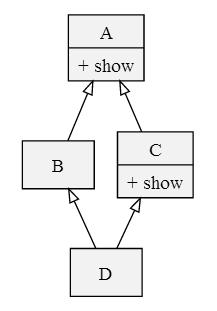Python 2.2 的新式类 MRO 计算方式和经典类 MRO 的计算方式非常相似：它仍然采用从左至右的深度优先遍历，但是如果遍历中出现重复的类，只保留最后一个。重新考虑上面「菱形继承」的例子：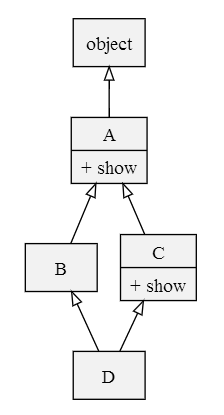import inspect

class A(object):pass
class B(A):pass
class C(A):pass
class D(B, C):pass

# 或者通过 D.__mro__ 查找
print inspect.getmro(D)


(<class '__main__.D'>,
<class '__main__.B'>,
<class '__main__.C'>,
<class '__main__.A'>,
<type 'object'>)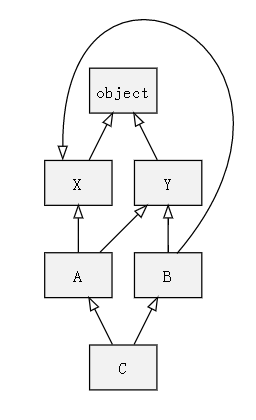1. 首先进行深度遍历：[C, A, X, object, Y, object, B, Y, object, X, object]；

2. 然后，只保留重复元素的最后一个：[C, A, B, Y, X, object]。

class X(object): pass
class Y(object): pass
class A(X, Y): pass
class B(Y, X): pass
class C(A, B): pass

print(C.__mro__)


Traceback (most recent call last):
File "F:/Python Script/Tornado/yang.py", line 7, in <module>
class C(A, B): pass
TypeError: Error when calling the metaclass bases
Cannot create a consistent method resolution
order (MRO) for bases X, Y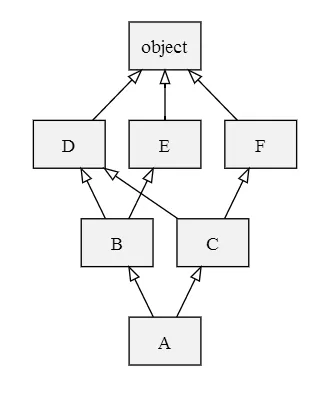1. 检查第一个列表的头元素（如 L[B1] 的头），记作 H。

2. 若 H 未出现在其它列表的尾部，则将其输出，并将其从所有列表中删除，然后回到步骤1；否则，取出下一个列表的头部记作 H，继续该步骤。

3. 重复上述步骤，直至列表为空或者不能再找出可以输出的元素。如果是前一种情况，则算法结束；如果是后一种情况，说明无法构建继承关系，Python 会抛出异常。

L[object] = [object]
L[D] = [D, object]
L[E] = [E, object]
L[F] = [F, object]
L[B] = [B, D, E, object]
L[C] = [C, D, F, object]
L[A] = [A] + merge(L[B], L[C], [B], [C])
= [A] + merge([B, D, E, object], [C, D, F, object], [B], [C])
= [A, B] + merge([D, E, object], [C, D, F, object], [C])
= [A, B, C] + merge([D, E, object], [D, F, object])
= [A, B, C, D] + merge([E, object], [F, object])
= [A, B, C, D, E] + merge([object], [F, object])
= [A, B, C, D, E, F] + merge([object], [object])
= [A, B, C, D, E, F, object]


class D(object): pass
class E(object): pass
class F(object): pass
class B(D, E): pass
class C(D, F): pass
class A(B, C): pass

A.__mro__


(<class '__main__.A'>,
<class '__main__.B'>,
<class '__main__.C'>,
<class '__main__.


## ## 附录：参考文章¶